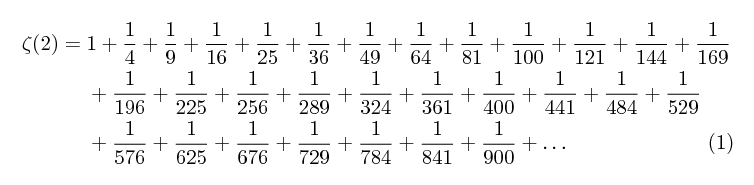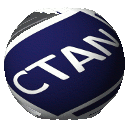# autobreak

This package implements a simple mechanism of line/page breaking within the align environment of the amsmath package; new line characters are considered as possible candidates for the breaks and the package tries to put breaks at adequate places. It is suitable for computer-generated long formulae with many terms.

## Example

\documentclass{article}
\usepackage{amsmath}
\usepackage{autobreak}
\pagestyle{empty}
\allowdisplaybreaks
\begin{document}

\begin{align}
\begin{autobreak}
\zeta(2) =
1
+ \frac{1}{4}
+ \frac{1}{9}
+ \frac{1}{16}
+ \frac{1}{25}
+ \frac{1}{36}
+ \frac{1}{49}
+ \frac{1}{64}
+ \frac{1}{81}
+ \frac{1}{100}
+ \frac{1}{121}
+ \frac{1}{144}
+ \frac{1}{169}
+ \frac{1}{196}
+ \frac{1}{225}
+ \frac{1}{256}
+ \frac{1}{289}
+ \frac{1}{324}
+ \frac{1}{361}
+ \frac{1}{400}
+ \frac{1}{441}
+ \frac{1}{484}
+ \frac{1}{529}
+ \frac{1}{576}
+ \frac{1}{625}
+ \frac{1}{676}
+ \frac{1}{729}
+ \frac{1}{784}
+ \frac{1}{841}
+ \frac{1}{900}
+ \dots
\end{autobreak}
\end{align}

\end{document}## Licence

The Project Public License 1.3 (or any later version)

## Bugs and remarks

Feedback is welcome on the Issue Tracker of GitHub:

https://github.com/tueda/autobreak/issues

 Package autobreak Version 0.3 2017-02-23 Licenses The LaTeX Project Public License 1.3 Maintainer Takahiro Ueda TDS archive autobreak.tds.zip Contained in TeX Live as autobreakMiKTeX as autobreak Topics Maths theoremMaths